Home > CCA2 > Chapter 10 > Lesson 10.3.2 > Problem10-177

10-177.
1. The sum of the digits of a two-digit number is 11, and when the digits are reversed the difference between the new number and the original is 27. Homework Help ✎

1. If you use t to represent the tens digit, and u to represent the units digit, how can you represent one of the numbers?

2. How can you represent the number with its digits reversed, still using t and u?

3. Use the information from the problem and parts (a) and (b) to write two equations that can be used to solve this problem.

4. What are the two numbers?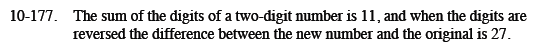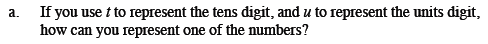10t + u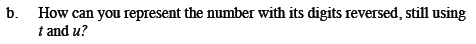See part (a). What effect does reversing the digits have?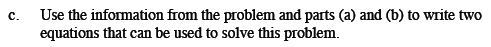How do you write an equation to find the sum of the digits?
How do you write an equation to find the differenece of the numbers?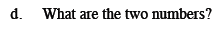74 and 47